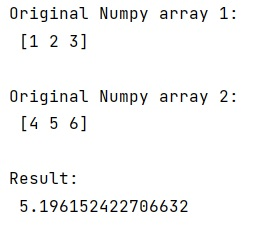# Calculate the Euclidean distance using NumPy

In this tutorial, we will learn how to calculate the Euclidean distance using NumPy? By Pranit Sharma Last updated : May 23, 2023

## What is Euclidean distance?

In Mathematics, the Euclidean distance is defined as the distance between two points in a given 2-dimensional space. In other words, the Euclidean distance between two points in Euclidean space is defined as the length of the line segment between two points.

If we have two points, such as (x1, y1) and (x2, y2) in the two-dimensional coordinate plane. Thus, the Euclidean distance formula is given by: d =√[(x2 – x1)2 + (y2 – y1)2]

Where,

• "d" is the Euclidean distance
• (x1, y1) is the coordinate of the first point
• (x2, y2) is the coordinate of the second point.

## How to Calculate the Euclidean distance using NumPy?

To calculate this distance using NumPy, the simplest method that you can use is numpy.linalg.norm(), it accepts the parameters with the default values like x, ord=None, axis=None, and keepdims=False. We can simply use this method by passing the two input NumPy array to calculate the Euclidean distance.

Let us understand with the help of an example,

## Example to Calculate the Euclidean distance using NumPy in Python

```# Import numpy
import numpy as np

# Creating two Numpy arrays
arr1 = np.array([1,2,3])
arr2 = np.array([4,5,6])

# Display original numpy arrays
print("Original Numpy array 1:\n",arr1,"\n")
print("Original Numpy array 2:\n",arr2,"\n")

# Finding euclidean distance
res = np.linalg.norm(arr1-arr2)

# Display result
print("Result:\n",res)
```

### Output

```Original Numpy array 1:
[1 2 3]

Original Numpy array 2:
[4 5 6]

Result:
5.196152422706632
```

### Output (Screenshot)